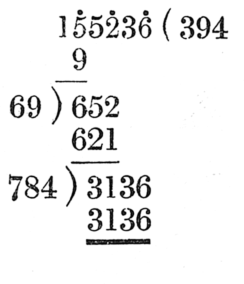#Square Roots

Math Lair Home > Topics > Square Roots

The square root of a number is a number which, when multiplied by itself, equals the given number. Each positive number has two square roots, one positive and one negative. For example, the square roots of 9 are 3 and −3. The positive root is referred to as the principal square root. The principal square root of a number is denoted by the symbol √ ; i.e. the principal square root of 9, which is 3, is denoted √9.

If the square root of a natural number is not equal to a natural number, then it is irrational. Either Pythagoras or another of the Pythagoreans who proved that 2 is irrational; in the fourth century B.C., Theatetus proved that the square roots of any integer that is not a square number are irrational.

The square root of 0 is 0. Negative numbers have no real square root, because there is no real number, whether negative or positive, that when multiplied by itself is negative. See complex numbers for further information about the square roots of negative numbers.

## Calculating Square Roots

Nowadays, when you need to calculate a square root, you'd probably reach for your calculator, computer, or other electronic device. There are several methods that you can use to calculate the first few digits of a square root of a number by hand or (sometimes) in your head.

One of these methods is called the Babylonian method. It was used by the Babylonians as well as some ancient Greek mathematicians such as Heron of Alexandria. Here's how it works:

Call N the number whose square root we want to find. Now, say that x is an estimate of √N. If x is an overestimate, then Nx is an underestimate, and vice versa. So, if we average x and Nx, then we should get a better estimate. This will be our new value of x, and we can repeat the process of averaging x and Nx until we get the required accuracy.

For example, say that we want to find the square root of 14. We could make an estimate of 4 for the value of the square root. 14 ÷ 4 = 72; the average of 4 and 72 is 154. We'll take 154 as our next estimate. 14 ÷ (15⁄4) = 5615. The average of 154 and 5615 is 449120. Up to this point I've done everything in my head (taking that last average was tricky, though) and I've achieved four-digit accuracy; 449120 is 3.7416, while √14 is 3.741657386... . If we wanted better accuracy, the process could be continued.

The square root of a number can also be calculated using a process similar to long division. This method is a little more complicated than the above method and isn't the sort of method you can do in your head. However, the above method has the weakness that, if you make a very poor estimate to begin with, it can take longer for the numbers to converge.The process is illustrated on the right, with 155,236 taken as an example. Divide the digits of the number up into groups of twos; you can separate each pair or, as shown in the example on the right, place a dot over the second digit of each pair. Look at the first pair of numbers, 15 in this case, and find the largest number whose square is no more than 15. This is 3, as 3² = 9, but 4² = 16. Write 3 to the right of the given number. This is the first digit of the answer. Subtract its square (9) from 15, giving 6.

Next, bring down the next pair of figures, 52. Take the answer so far, 3, and multiply it by 2, giving 6. Write that to the left of 652. Now, find the largest number, x, such that x(60 + x) is no more than 652. By trial and error, it turns out that 9 × 69 = 621. So, 9 will be the second digit of our answer; write it next to the 3. Subtract 621 from 652, leaving 21.

Next, bring down the next pair of figures, 36, and repeat the same process. 39 × 2 = 78; the largest x such that x(780 + x) is no more than 3136 is 4; write 4 as the next digit of the answer and subtract 784 × 4 = 3136 from 3136; the result is 0, so the square root is exact. The answer is 394.

In older books you may see the term "evolution" used to describe finding the roots of given numbers.